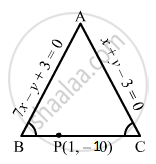Advertisement Remove all ads

# Two Sides of an Isosceles Triangle Are Given by the Equations 7x − Y + 3 = 0 and X + Y − 3 = 0 and Its Third Side Passes Through the Point (1, −10). Determine the Equation of the Third Side. - Mathematics

Answer in Brief

Two sides of an isosceles triangle are given by the equations 7x − y + 3 = 0 and x + y − 3 = 0 and its third side passes through the point (1, −10). Determine the equation of the third side.

Advertisement Remove all ads

#### Solution

Let ABC be the isosceles triangle, where 7x − y + 3 = 0 and x + y − 3 = 0 represent the sides AB and AC, respectively.
Let AB = BC$\because$ AB = BC

$\therefore$ tan B = tan C
Here,
Slope of AB = 7
Slope of AC = −1
Let m be the slope of BC.

$\text { Then,} \left| \frac{m - 7}{1 + 7m} \right| = \left| \frac{m + 1}{1 - m} \right| = \left| \frac{m + 1}{m - 1} \right|$

$\Rightarrow \frac{m - 7}{1 + 7m} = \pm \frac{m + 1}{m - 1}$

Taking the positive sign, we get:

$m^2 - 8m + 7 = 7 m^2 + 8m + 1$

$\Rightarrow \left( m + 3 \right)\left( m - \frac{1}{3} \right) = 0$

$\Rightarrow m = - 3, \frac{1}{3}$

Now, taking the negative sign, we get:

$\left( m - 7 \right)\left( m - 1 \right) = - \left( 7m + 1 \right)\left( m + 1 \right)$

$\Rightarrow m^2 - 8m + 7 = - 7 m^2 - 8m - 1$

$\Rightarrow m^2 = - 1\text { (not possible) }$

Equations of the third side is

$y + 10 = - 3\left( x - 1 \right) \text { and } y + 10 = \frac{1}{3}\left( x - 1 \right)$

$\Rightarrow 3x + y + 7 = 0 \text { and } x - 3y - 31 = 0$

Concept: Straight Lines - Equation of Family of Lines Passing Through the Point of Intersection of Two Lines
Is there an error in this question or solution?
Advertisement Remove all ads

#### APPEARS IN

RD Sharma Class 11 Mathematics Textbook
Chapter 23 The straight lines
Exercise 23.18 | Q 10 | Page 125
Advertisement Remove all ads
Advertisement Remove all ads
Share
Notifications

View all notifications

Forgot password?